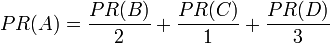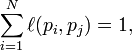mokotw

什麼是Comment Spam?

Posted in what 由 mokotw 於 二月 14, 2007

什麼是SPAM ？哪些網頁會被認定為SPAM ？

Posted in what,yahoo 由 mokotw 於 二月 14, 2007

什麼是PageRank

Posted in what 由 mokotw 於 一月 29, 2007

PageRank演算法

PageRank讓連結來"投票"

PageRank演算法中的點擊演算法是由Jon Kleinberg提出的.

PageRank演算法

PR(A) = PR(B) + PR(C) + PR(D)$PR(A)= \frac{PR(B)}{2}+ \frac{PR(C)}{1}+ \frac{PR(D)}{3}$$PR(A)= \frac{PR(B)}{L(B)}+ \frac{PR(C)}{L(C)}+ \frac{PR(D)}{L(D)}$$PR(A)=\left( \frac{PR(B)}{L(B)}+ \frac{PR(C)}{L(C)}+ \frac{PR(D)}{L(D)}+\,\cdots \right) q + 1 - q$

完整的${\rm PageRank}(p_i) = \frac{q}{N} + (1 -q) \sum_{p_j} \frac{{\rm PageRank} (p_j)}{L(p_j)}$

p1,p2,…,pN是被研究的頁面, M(pi)是鏈入pi頁面的數量, L(pj)pj鏈出頁面的數量, 而N是所有頁面的數量。

PageRank值是一個特殊矩陣中的特徵向量。這個特徵向量為$\mathbf{R} = \begin{bmatrix} {\rm PageRank}(p_1) \\ {\rm PageRank}(p_2) \\ \vdots \\ {\rm PageRank}(p_N) \end{bmatrix}$

R是等式的答案$\mathbf{R} = \begin{bmatrix} {q / N} \\ {q / N} \\ \vdots \\ {q / N} \end{bmatrix} + (1-q) \begin{bmatrix} \ell(p_1,p_1) & \ell(p_1,p_2) & \cdots & \ell(p_1,p_N) \\ \ell(p_2,p_1) & \ddots & & \\ \vdots &amp;amp; & \ell(p_i,p_j) & \\ \ell(p_N,p_1) & & & \ell(p_N,p_N) \end{bmatrix} \mathbf{R}$$\sum_{i = 1}^N \ell(p_i,p_j) = 1,$

「PageRank」的文獻信息

QR Code (Quick Response Code)

Posted in what 由 mokotw 於 一月 28, 2007什麼是網路上的﻿”點閱詐欺﻿”?

Posted in what 由 mokotw 於 一月 28, 2007

CNET新聞專區：Stefanie Olsen報導 7/3/2005
（唐慧文） http://taiwan.cnet.com/news/softwar…20096986,00.htm

「這是為數可能高達十億美元的問題，」新興搜尋引擎公司Snap.com總裁Tom McGovern說。

Alchemist Media創辦人兼點閱詐欺專家Jessie Stricchiola說，通常，對涉嫌詐欺的人士寄發「勒令停止通知函」(cease-and-desist letter)，即可收到嚇阻之效。對2KMedical的Harrison來說，這類警告函亦可引起第二家搜尋引擎的注意，以遏制對手公司的不法行徑。